# Ratio To Fraction Worksheet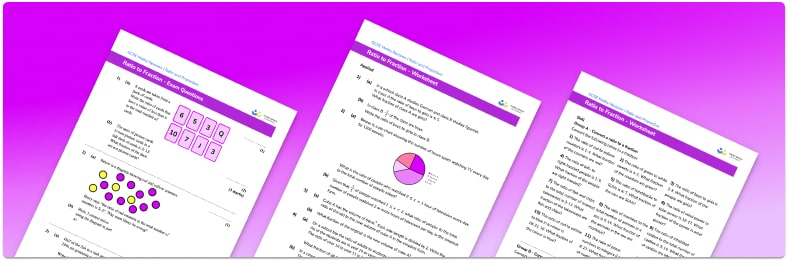• This field is for validation purposes and should be left unchanged.

You can unsubscribe at any time (each email we send will contain an easy way to unsubscribe). To find out more about how we use your data, see our privacy policy.

• Section 1 of the ratio to fraction worksheet contains 36 skills-based ratio to fraction questions, in 3 groups to support differentiation
• Section 2 contains 4 applied ratio to fraction questions with a mix of worded problems and deeper problem solving questions
• Section 3 contains 4 foundation and higher level GCSE exam style simplifying and equivalent ratio to fraction questions
• Answers and a mark scheme for all ratio to fraction questions are provided
• Questions follow variation theory with plenty of opportunities for students to work independently at their own level
• All questions created by fully qualified expert secondary maths teachers
• Suitable for GCSE maths revision for AQA, OCR and Edexcel exam boards

### Ratio to fraction at a glance

A ratio compares how much there is of one thing in relation to another. If 3 people are left handed and 7 people are right handed then the ratio of left to right handed people is 3:7.

We can convert ratios to fractions. The total number of people in the ratio above is 3+7=10. The denominator of the fraction is the total of the parts in the ratio, the numerator is one part of the ratio. This means that 3 out of the 10 people are left handed, and 7 out of the 10 people are right handed.

Any fraction or ratio should contain integers (whole numbers) when written in its simplest form.

Looking forward, students can then progress to additional ratio and proportion worksheets, for example aor a direct proportion worksheet.

For more teaching and learning support on Ratio and Proportion our GCSE maths lessons provide step by step support for all GCSE maths concepts.

## Related worksheets

Simplifying Ratios Worksheet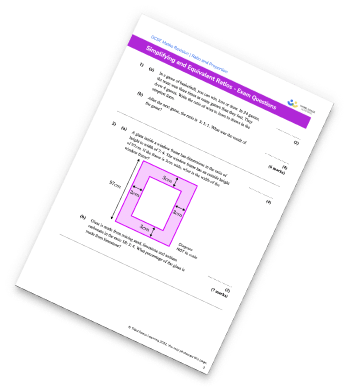Ratio Worksheet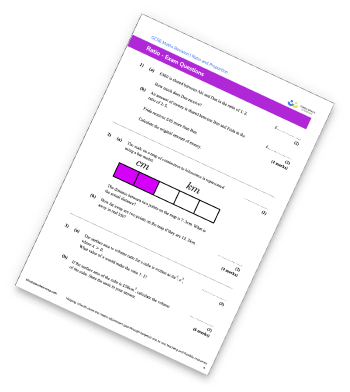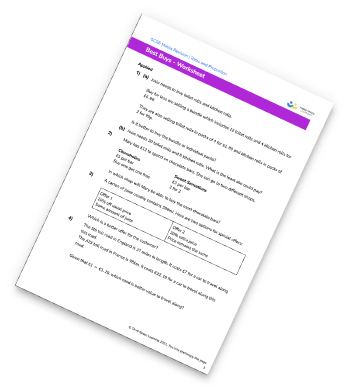Proportion Worksheet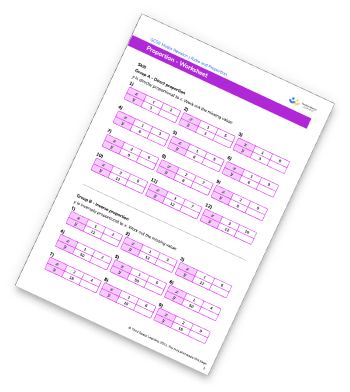## Do you have KS4 students who need more focused attention to succeed at GCSE?There will be students in your class who require individual attention to help them succeed in their maths GCSEs. In a class of 30, it’s not always easy to provide.

Help your students feel confident with exam-style questions and the strategies they’ll need to answer them correctly with our dedicated GCSE maths revision programme.

Lessons are selected to provide support where each student needs it most, and specially-trained GCSE maths tutors adapt the pitch and pace of each lesson. This ensures a personalised revision programme that raises grades and boosts confidence.

Find out more